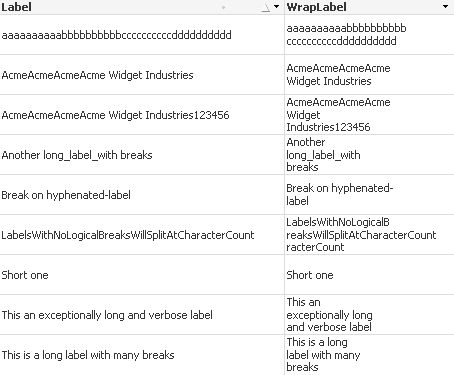# QlikView Layout & Visualizations

Discussion Board for collaboration on QlikView Layout & Visualizations.

Announcements
QlikWorld, June 24-25, 2020. Free virtual event for DI and DA gurus. Register Today
cancel
Showing results for
Did you mean:
HighlightedMaster III

## Re: RE:Size Reduce

Try this

try this...

=replace(field name,' ',chr(13)&' ')

HighlightedMVP & Luminary

## Re: RE:Size Reduce

Hi,

The best option for this scenario is use horizontal orientation.

Regards,

Jagan.

HighlightedMVP

## Re: RE:Size Reduce

It is a little complex to break intelligently on spaces or hyphens, but here some code that you could incorporate into your load after you have loaded the table containing the label to split. Assuming the table is named Data and the field is called Label:

Set vBreak = 20;  // maximum length

WrapLabel:

If(t1 = 0 And t2 = 0 And ln <= \$(vBreak),

Label,

If(t1 = 0 And t2 = 0 And ln > \$(vBreak) And ln <= \$(vBreak) * 2,

Left(Label, \$(vBreak)) & chr(10) & Mid(Label, \$(vBreak) + 1, 999),

If(t1 = 0 And t2 = 0 And ln > \$(vBreak) * 2,

Left(Label, \$(vBreak)) & chr(10) & Mid(Label, \$(vBreak) + 1, \$(vBreak)*2 - 1) & chr(10) & Mid(Label, \$(vBreak)*2, 999),

If(t1 > 0 And t2 <= t1,

Left(Label, t1) & chr(10) & Mid(Label, t1 + 1, 999),

If(t2 > t1,

Left(Label, t1) & chr(10) & Mid(Label, t1 + 1, t2 - t1 -1) & chr(10) & Mid(Label, t2 + 1, 999)

))))) As WrapLabel

;

If((t1 > 0) And (ln - t1 > \$(vBreak)), Index(Mid(st, t1 + 1, \$(vBreak)), ' ', -1) + t1, 0) As t2

;

If(ln > \$(vBreak), Index(Left(st, \$(vBreak)), ' ', -1), 0) As t1

;

Len(Label) As ln,

Replace(Label, '-', ' ') As st

;

Label

Resident Data;

This can remain as a separate table and you can use WrapLabel in place of Label as the chart dimension. Adapt the script to your table and field names. Set vBreak to a suitable break size.

EDIT - on further testing, found a bug with the fixed wrap which is now fixed.Logic will get you from a to b. Imagination will take you everywhere. - A Einstein
HighlightedChampion III

## Re: RE:Size Reduce

nice jonathan..

HighlightedChampion III

## Re: RE:Size Reduce

hi settu ,

small changes to ur expression .got the olution wat i required.

=left(Account, findoneof(Account, ' ', ceil(substringcount(Account, ' ')/2))) & chr(13) & chr(10) & right(Account, len(Account) - findoneof(Account, ' ', ceil(substringcount(Account, ' ')/2)))

thanks

Suresh